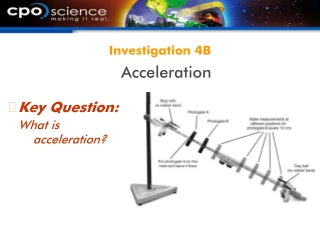DownloadDownload PresentationInvestigation 4B

# Investigation 4B

Télécharger la présentation## Investigation 4B

- - - - - - - - - - - - - - - - - - - - - - - - - - - E N D - - - - - - - - - - - - - - - - - - - - - - - - - - -
##### Presentation Transcript

1. Key Question: What is acceleration? Investigation 4B Acceleration

2. Accelerationis the rate at which your speed (or velocity) changes. 4.3 Acceleration • If your speed increases by 1 meter per second (m/s) for each second, then your acceleration is 1 m/s per second.

3. There is zero acceleration at constant speed because the speed does not change. 4.3 Acceleration

4. Acceleration is easy to spot on a speed vs. time graph. 4.3 Acceleration • Acceleration causes the line to slope up on a speed vs. time graph. What is the bike’s acceleration?

5. If the hill is steeper, the acceleration is greater. 4.3 Acceleration

6. Speed and acceleration are not the same thing. You can be moving (constant speed), but have no acceleration (think cruise control). 4.3 Acceleration

7. Acceleration describes how quickly speed changes. Acceleration is the change in speed divided by the change in time. 4.3 Acceleration

8. An acceleration of 20 km/h/s means that the speed increases by 20 km/h each second. The units for time in acceleration are often expressed as “seconds squared” and written as s2. 4.3 Speed and acceleration Can you convert this rate to m/s2 using conversion factors?

9. Solving Problems • A sailboat moves at 1 m/s. • A strong wind increases its speed to 4 m/s in 3 s. • Calculate acceleration.

10. Solving Problems • Looking for: • …acceleration of sailboat • Given: • …v1 = 1 m/s; v2 = 4 m/s; time = 3 s • Relationships: • a = v2 – v1/t • Solution: • a = (4 m/s – 1 m/s)/ 3 s = 1 m/s2

11. The word “acceleration” is used for any change in speed, up or down. Acceleration can be positive or negative. 4.3 Acceleration on motion graphs

12. Positive acceleration adds more speed each second. Things get faster. Speed increases over time. 4.3 Acceleration on speed-time graphs

13. Negative acceleration subtracts some speed each second. Things get slower. People sometimes use the word deceleration to describe slowing down. 4.3 Acceleration on speed-time graphs

14. The position vs. time graph is a curve when there is acceleration. The car covers more distance each second, so the position vs. time graph gets steeper each second. 4.3 Acceleration on position-time graphs

15. When a car is slowing down, the speed decreases so the car covers less distance each second. The position vs. time graph gets shallower with time. 4.3 Acceleration on position-time graphs

16. An object is in free fall if it is accelerating due to the force of gravity and no other forces are acting on it. 4.3 Free fall

17. 4.3 Free fall • The letter “g” is used for acceleration due to gravity. • Falling objects increase their speed by 9.8 m/s every second, or 9.8 m/s2

18. 4.3 Acceleration and direction • Acceleration occurs whenever there is a change in speed, direction, or both.

19. 4.3 Acceleration and direction • A car driving around a curve at a constant speed is accelerating because its direction is changing.

20. 4.3 Acceleration and direction • Individual vectors can be drawn to scale to calculate the change in direction.

21. 4.3 Curved motion • A soccer ball is an example of a projectile. • A projectile is an object moving under the influence of only gravity. • The path of the ball makes a bowl-shaped curve called a parabola.

22. 4.3 Curved motion • Circular motion is another type of curved motion. • An object in circular motion has a velocity vector that constantly changes direction.

23. Key Question: What happens to the Energy Car as it travels down a hill and across a flat section of track? Investigation 4C Studying Two Part Motion

24. High Tech Animal Trackers • The more we learn about how animals interact with their environments, the better decisions we can make about how we use the oceans. • Satellite tagging research studies have led to many new laws and guidelines governing human activities around endangered species.